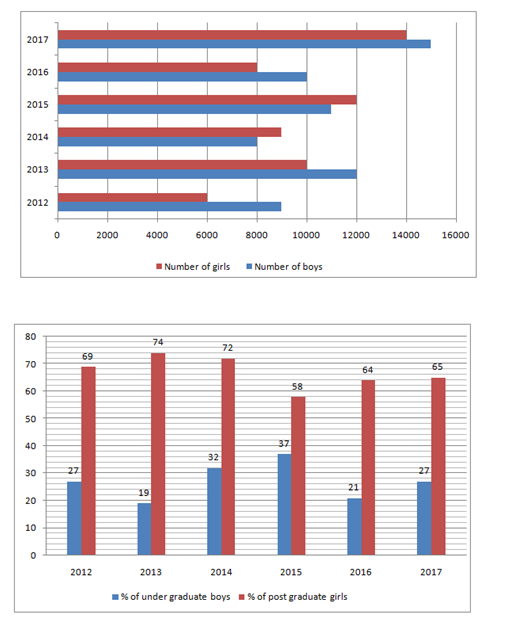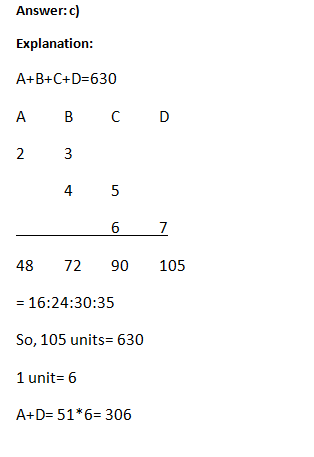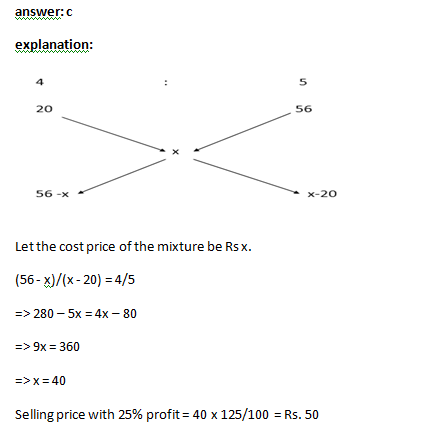# “20-20” Quantitative Aptitude | Crack SBI Clerk 2018 Day-27

Dear Friends, SBI Clerk 2018 Notification has been released we hope you all have started your preparation. Here we have started New Series of Practice Materials specially for SBI Clerk 2018. Aspirants those who are preparing for the exams can use this “20-20” Quantitative Aptitude Questions.

## Daily Practice Test Schedule | Good Luck

 Topic Daily Publishing Time Daily News Papers & Editorials 8.00 AM Current Affairs Quiz 9.00 AM Logical Reasoning 10.00 AM Quantitative Aptitude “20-20” 11.00 AM Vocabulary (Based on The Hindu) 12.00 PM Static GK Quiz 1.00 PM English Language “20-20” 2.00 PM Banking Awareness Quiz 3.00 PM Reasoning Puzzles & Seating 4.00 PM Daily Current Affairs Updates 5.00 PM Data Interpretation / Application Sums (Topic Wise) 6.00 PM Reasoning Ability “20-20” 7.00 PM English Language (New Pattern Questions) 8.00 PM General / Financial Awareness Quiz 9.00 PM

“20-20” Aptitude Questions | Crack SBI Clerk 2018 Day-27

Click “Start Quiz” to attend these Questions and view Explanation

### Click Here for SBI Clerk Prelims and Mains 2018 – Full Length Mock Test

Directions (Q. 1 -5): What Approximate value should come in place of question mark (?) in the following questions.

1). 820.01 ÷ 21 × 2.99 + ? = 240

1. 105
2. 173
3. 123
4. 234
5. 143

2). 299 ÷ 12 × 13.95 + ? = (24.02)2

1. 285
2. 225
3. 325
4. 150
5. 185

3). 92 × 576 ÷  √1296 = (?)3 + √49

1. 3
2. 14
3. 11
4. 27
5. None of these
1. (21.5% of 999)^(1/3) + (43% of 601)^1/2 = ?
1. 18
2. 22
3. 26
4. 30
5. None of these
1. 40.005% of 439.998 + ? % of 655.011 = 228.5
1. 8
2. 17
3. 12
4. 20
5. 5

Direction (Q. 6-10): What will come in the place of question mark (?) in the following number series?

1. 45, 57,   67,   75,   81,   ?
1. 89
2. 85
3. 105
4. 91
5. 78
1. 5, 7.5,   15,   37.5,  ? ,   393.75
1. 80
2. 112.5
3. 160
4. 48
5. 72

8). 5,   20,   53,   112,   205,   ?

1. 320
2. 330
3. 340
4. 350
5. None of these

9). 20,   20,   10,   30,   7.5,   ?

1. 32.5
2. 37.5
3. 41.25
4. 43.25
5. None of these

10). 64,   66,   72,   88,   128,   ?

1. 256
2. 246
3. 224
4. 226
5. None of these
1. The sum of four number is 630. If the ratio of the first and second number is 2:3, ratio of second and third number is 4:5 and the ratio of the third and fourth number is 6:7, then find the sum of first and last number?
1. 287
2. 564
3. 306
4. 215
5. None of these
1. Bag A contains 5 red, 4 green and 3 blue balls, bag B contains 7 red, 3 green and 5 blue balls. Three balls are drawn at random from each bag. Find the ratio of the probabilities that balls drawn from each of the bags are of different colours.
1. 11:12
2. 12:11
3. 13:11
4. 11:15
5. None of these
1. Ravi spent 40% of his salary on food, 25% of the remaining on clothes, 35% of the remaining on miscellaneous expenditure and he saves Rs. 58500. Find the average amount he spent on food and clothes?
1. Rs. 45000
2. Rs. 44000
3. Rs. 55000
4. Rs. 35000
5. none of these
1. A shopkeeper mixes two types of rice costing Rs.20 and Rs.56 in the ratio 4:5 respectively. What should be the selling price of the mixture, if the shopkeeper wants to earn a profit of 25%?
1. Rs.30
2. Rs.40
3. Rs.50
4. Rs.45
5. None of these
1. The ratio of present age of Kavitha to that Sunitha is 4:13. Chandra is 15 years older than Sunitha. Chandra’s age after 8 years will be 75 years. What is the present age of kavitha’s mother, who is 30 years older than Kavitha?
1. 46 years
2. 43 years
3. 78 years
4. 67 years
5. None of these

Directions (Q. 16-20): Study the following information carefully and answer the questions given below.

Following bar-graph shows the total number of boys and girls selected in IBPS examination during the period 2012 to 2017 and the column graph shows the percentage of under graduate boys among total boys and the percentage of Post graduate girls among total girls selected in examination.

Note: Graduation is the qualification for the exam1. In the year 2016, the total number of boys selected from Under graduate stream is what percentage of the total number of girls selected from Post graduate stream? (Find approximate value only.)
1. 30%
2. 35%
3. 41%
4. 49%
5. 45%

1. In the year 2015, the number of boys selected from Post graduate stream is what percentage more than the number of boys selected from Under graduate stream?
1. 70.27%
2. 63.54%
3. 73.54%
4. 60.92%
5. 75.84%
1. What is the ratio of the girls selected from Post graduate stream in the year 2012 to the number of girls selected from Under graduate stream in the year 2016?
1. 10 : 7
2. 19 : 12
3. 22 : 15
4. 24 : 17
5. 23 : 16
1. What is the difference between the total number of boys selected from Post graduate stream and the total number of girls selected from Post graduate stream in all the six years together?
1. 8570
2. 8090
3. 8250
4. 8310
5. 8010
1. What is the average number of girls selected from Under graduate stream in all six years?
1. 3000
2. 3300
3. 3900
4. 3100
5. 3500

Directions (Q. 1 -5):

Explanation:

820 ÷ 21 × 3 + ? ≈ 240

Or, 39 × 3 + ? ≈ 240

Or, ? ≈ 240 – 117 = 123

Explanation:

? ≈ (24.02)2 – 299 ÷ 12 × 13.95

≈ 576 – 25 × 14 = 576 – 350 = 226 ≈ 225

Explanation:

(?)3 + √49 = 92 × 876 ÷ √1296

(?)3 + 7 = 92 × 576 ÷ 36

(?)3 + 7 = 92 × 576 ÷36

(?)3 = 1465

?= 11.3

Explanation:

? = (21.5 * 10)^1/3 + (43 * 6)^1/2

? = (215)^(1/3) + (258)^(1/2)

? = (6^3)^(1/3) + (16^2)^(1/2)

? = 6 + 16 = 22

Explanation:

40 * (440/100) + ?/100 * 655 = 228

4*44 + ? * 6.55 = 229

? = (229 – 176) / 7

? = 53/7 = 7.5 = 8

Direction (Q. 6-10):

Explanation:

The series is

45 + 12 = 57;

57 + 10 = 67;

67 + 8 = 75;

75 + 6 = 81;

81 + 4 = 85

Explanation:

The series is

5 × 1.5 = 7.5;

7.5 × 2 = 15;

15 × 2.5 = 37.5;

37.5 × 3 = 112.5;

112.5×3.5 = 393.75

Explanation:

5 + (4^2 – 1) = 20

20 + (6^2 -3) = 53

53 + (8^2 -5) = 112

112 + (10^2 – 7) = 205

205 +(12^2 – 9) = 340

Explanation:

20*1 = 20

20 ÷ 2 = 10

10 * 3 = 30

30 ÷4 = 7.5

7.5 * 5 = 37.5

Explanation:

64 + (1*2) = 66

66 + (2*3) = 72

72 + (4*4) = 88

88 + (8*5) = 128

128 + (16*6) = 224

11.explanation:

probability of balls drawn from bag A are of different colours = (5c1 x 4c1 x 3c1)/12c3

= (5 x 4 x 3)/220 = 3/11

probability of balls drawn from bag B are of different colours = (7c1 x 3c1 x 5c1)/15c3

= (7 x 3 x 5)/455 = 3/13

Required ratio: 3/11 : 3/13 = 13:11

explanation:

salary = 100/(100-40) x 100/(100-25) x 100/(100-35) x 58500 = Rs.200000

spending on food = 200000 x 40/100 = 80000

spending on clothes = 200000 x 60/100 x 25/100 = 30000

sum of spending on food and cloth together = 80000 + 30000 = 110000

average = 110000/2 =Rs. 55000

14.Explanation:

According to the question,

Chandra’s present age = 75 – 8 = 67 years

Hence, Sunitha’s age = 67 – 15 = 52 years

∴ The age of Kavitha = 4/13 × 52 = 16 years

Hence, Kavitha’s mother’s age = 16 + 30 = 46 years.

Directions (Q. 16-20):

Explanation:

Under graduate Boys = 10000 × 21/100

= 2100

Post graduate Girls = 8000 × 64/100

= 5120

Required % = 2100/5120 × 100

= 41%

Explanation:

Required % = (63 – 37)/37 × 100

= 2600/37

= 70.27%

Explanation:

Post graduate Girls 2012 = 6000 × 69/100

= 4140

Under graduate Girls 2016 = 8000 × 36/100

= 2880

Ratio = 414/288

= 23/16

= 23 : 16

Explanation:

Total no of Post graduate Boys = 6570+9720+5440+6930+7900+10950 = 47510

Total no of Post graduate Girls = 4140+7400+6480+6960+5120+9100 = 39200

Difference = 47510 – 39200

= 8310